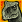## Fraction data convert to Float

cancel
Showing results for
Did you mean:
SOLVED

## Fraction data convert to Float

729 ViewsContributor III

Fraction data convert to Float

I have result fraction data format with below Math_Lib

But that is the data type difficult show to me

So, i want to show "0x10000000(FRAC32)" --> "0.125"

How can i do?

#include "mlib.h"

tFrac32 f32In1;

tFrac32 f32In2;

tFrac32 f32Out;

Function MLIB_Mul_F32

Automotive Math and Motor Control Library Set for Carcassonne MC9S12ZVM devices, Rev. 8

450 Freescale Semiconductor, Inc.

void main(void)

{

// first input = 0.5

f32In1 = FRAC32 (0.5);

// second input = 0.25

f32In2 = FRAC32 (0.25);

// output should be 0x10000000 = FRAC32(0.125)

f32Out = MLIB_Mul_F32(f32In1,f32In2);

// output should be 0x10000000 = FRAC32(0.125)

f32Out = MLIB_Mul (f32In1,f32In2,F32);

// ##############################################################

// Available only if 32-bit fractional implementation selected

// as default

// ##############################################################

// output should be 0x10000000 = FRAC32(0.125)

f32Out = MLIB_Mul (f32In1,f32In2);

Labels (1)
• ### General

1 Solution
235 ViewsSenior Contributor IV

0x10000000 / 0x80000000 = 0.125, so for unsigned fractions conversion could look like this

float f = (float)x / 0x80000000 ;

Signed:

if( x & 0x8000000) // is it negative

f = - ( (float)(0-x) / 0x80000000 );

else

f = (float)x / 0x80000000 ;

2 Replies
236 ViewsSenior Contributor IV

0x10000000 / 0x80000000 = 0.125, so for unsigned fractions conversion could look like this

float f = (float)x / 0x80000000 ;

Signed:

if( x & 0x8000000) // is it negative

f = - ( (float)(0-x) / 0x80000000 );

else

f = (float)x / 0x80000000 ;

235 ViewsNXP Employee

Hi Ahn,

As correctly mentioned by Edward:

We should divide 32-bit fixed point number in format Q1.31 by 0x80000000 for getting signed fractional [-1,1) number.

FRAC32() is defined in SWLIBS_Defines.h file as:

`/*! Macro converting a signed fractional [-1,1) number into a 32-bit fixed point number in format Q1.31.*/#define FRAC32(x) ((tFrac32) ((x < FRACT_MAX) ? ((x >= FRACT_MIN) ? (x*2147483648.0) : INT32_MIN) : INT32_MAX))`

Note: 2147483648=0x80000000

Since tFrac32 type = tS32 type = signed long type (according SWLIBS_Typedefs.h), we do not need to test sign in this case and command

`float f = (float)x / 0x80000000;`

should work correctly also for negative numbers.

Code

`if( x & 0x8000000) // is it negative    f = - ( (float)(0-x) / 0x80000000 );else    f = (float)x / 0x80000000 ;`

is necessary when input data x are unsigned type.

I hope it helps you.

Have a great day,